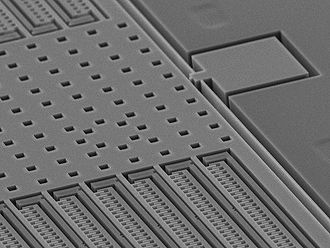MEMS is typically the field in which a true multiphysical modeling is needed. Indeed, all physical phenomena are strongly coupled (from the mathematical point of view). Therefore, you need to use specific tools to take that into consideration.

With Comsol Multiphysics, we can solve modeling problems, including as many coupled phenomena as needed, with a strong solver to avoid iterative numerical scheme between equation. It can make the difference !

• electromagnetism with Coulomb forces (virtual work or with the Maxwell tensor)

• structural mechanics to compute the displacement of the device

• microfluidics, with wetted wall and contact angles